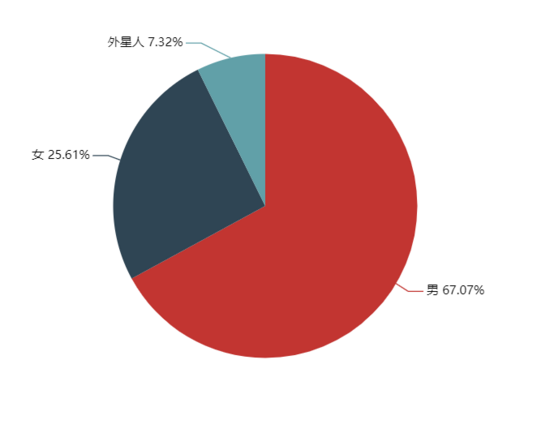# Python获取好友地区分布及好友性别分布情况代码详解

更新时间：2019年07月10日 11:26:51   作者：故事胶片我要评论

python + wxpy 机器人

1. 编辑器
2. 一个注册一年以上的微信号

```from wxpy import * // wxpy 依赖
from PIL import Image  // 二维码登录依赖
import os  // 本地下载依赖
import math
import webbrowser
from pyecharts import Map  // 地图展示依赖
from pyecharts import Pie  // 饼状图依赖```

1. 批量下载好友头像

```# 创建头像存放文件夹
def avaterPath():
avaterDir = os.path.join(os.getcwd(), 'wechat')
if not os.path.exists(avaterDir):
os.mkdir(avaterDir)
return avaterDir

# 获取所有的好友头像并保存
def saveWxAvater(avaterDir):
bot = Bot(cache_path=True)
allFriends = bot.friends(update=True)
num = 0
for friend in allFriends:
friend.getAvatar(os.path.join(avaterDir,f'{str(num)}.jpg'))
print("好友昵称：%s"%friend.name)

num += 1

# 拼接头像
def joinAvatar(path):
# 获取文件夹内头像个数
length = len(os.listdir(path))
# 设置画布大小
image_size = 2560
# 设置每个头像大小
each_size = math.ceil(2560 / math.floor(math.sqrt(length)))
# 计算所需各行列的头像数量
x_lines = math.ceil(math.sqrt(length))
y_lines = math.ceil(math.sqrt(length))
image = Image.new('RGB', (each_size * x_lines, each_size * y_lines))
x = 0
y = 0
for (root, dirs, files) in os.walk(path):
for pic_name in files:
try:
with Image.open(os.path.join(path, pic_name)) as img:
img = img.resize((each_size, each_size))
image.paste(img, (x * each_size, y * each_size))
x += 1
if x == x_lines:
x = 0
y += 1
except Exception as e:
print(F"头像读取失败，错误：{e}")

img = image.save(os.path.join(os.getcwd(), 'wechat.png'))
print('wx好友头像拼接完成!')

if __name__ == '__main__':
avatarDir = avaterPath()
saveWxAvater(avatarDir)
joinAvatar(avatarDir)```

2. 获取好友性别分布

```bot = Bot(cache_path=True) # 弹出二维码登录微信，生成bot对象

allFriends = bot.friends() # 获取所有的微信好友信息

type = ['男同学','女同学','外星人'] # 男/女/未知性别好友名称

v = [0, 0, 0] # 初始化对象好友数量

# 遍历所有好友，判断该好友性别
for friend in friends:
if friend.sex == 1:
v += 1
elif friend.sex == 2:
v += 1
else:
v += 1

pie = Pie("好友性别分布")

pie.render("sex.html")

webbrowser.open('sex.html')```3. 获取好友地区分布情况

```bot = Bot(cache_path=True) # 弹出二维码登录微信，生成bot对象

allFriends = bot.friends() # 获取所有的微信好友信息

for friend in allFriends:
else:

keys = area_dic.keys()
v = area_dic.values()

map = Map("好友地域分布", width=1200, height=600)